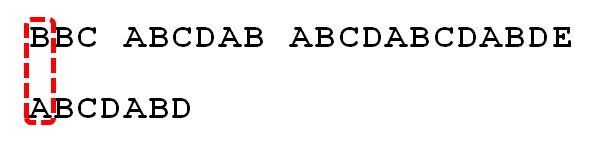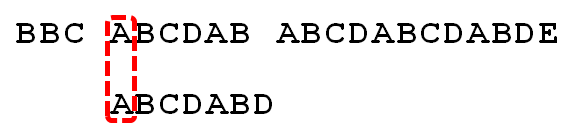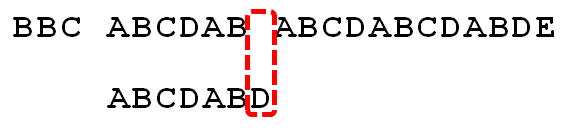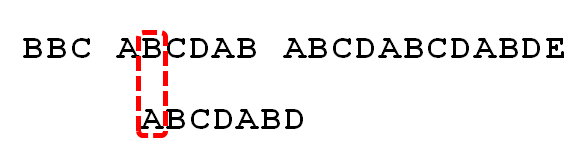# 2. 暴力匹配算法

• 如果当前字符匹配成功（即 S[i] == P[j]），则 i++，j++，继续匹配下一个字符；
• 如果失配（即 S[i]! = P[j]），令 i = i - (j - 1)，j = 0。相当于每次匹配失败时，i 回溯，j 被置为0。

``````int ViolentMatch(char* s, char* p)
{
int sLen = strlen(s);
int pLen = strlen(p);

int i = 0;
int j = 0;
while (i < sLen && j < pLen)
{
if (s[i] == p[j])
{
//①如果当前字符匹配成功（即S[i] == P[j]），则i++，j++
i++;
j++;
}
else
{
//②如果失配（即S[i]! = P[j]），令i = i - (j - 1)，j = 0
i = i - j + 1;
j = 0;
}
}
//匹配成功，返回模式串p在文本串s中的位置，否则返回-1
if (j == pLen)
return i - j;
else
return -1;
}  ``````

1.S 为 B，P 为 A，不匹配，执行第 ② 条指令：“如果失配（即 S[i]! = P[j]），令 i = i - (j - 1)，j = 0”，S 跟 P 匹配，相当于模式串要往右移动一位（i=1，j=0）2.S 跟 P 还是不匹配，继续执行第 ② 条指令：“如果失配（即 S[i]! = P[j]），令 i = i - (j - 1)，j = 0”，S 跟 P 匹配（i=2，j=0），从而模式串不断的向右移动一位（不断的执行“令 i = i - (j - 1)，j = 0”，i 从 2 变到 4，j 一直为 0）3.直到 S 跟 P 匹配成功（i=4，j=0），此时按照上面的暴力匹配算法的思路，转而执行第 ① 条指令：“如果当前字符匹配成功（即 S[i] == P[j]），则 i++，j++”，可得 S[i] 为 S，P[j] 为 P，即接下来 S 跟 P 匹配（i=5，j=1）4.S 跟 P 匹配成功，继续执行第 ① 条指令：“如果当前字符匹配成功（即 S[i] == P[j]），则 i++，j++”，得到 S 跟 P 匹配（i=6，j=2），如此进行下去5.直到 S 为空格字符，P 为字符 D（i=10，j=6），因为不匹配，重新执行第 ② 条指令：“如果失配（即 S[i]! = P[j]），令 i = i - (j - 1)，j = 0”，相当于 S 跟 P 匹配（i=5，j=0）6.至此，我们可以看到，如果按照暴力匹配算法的思路，尽管之前文本串和模式串已经分别匹配到了 S、P，但因为 S 跟 P 不匹配，所以文本串回溯到 S，模式串回溯到 P，从而让 S 跟 P 匹配。